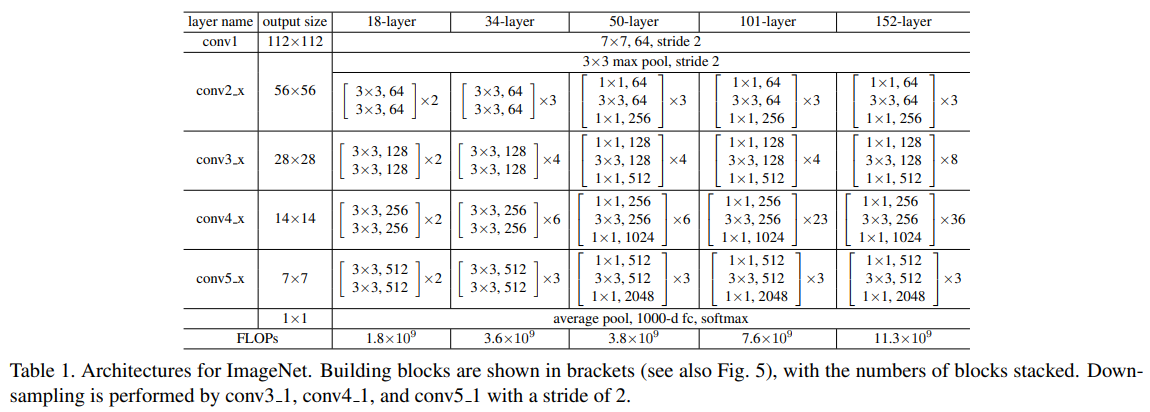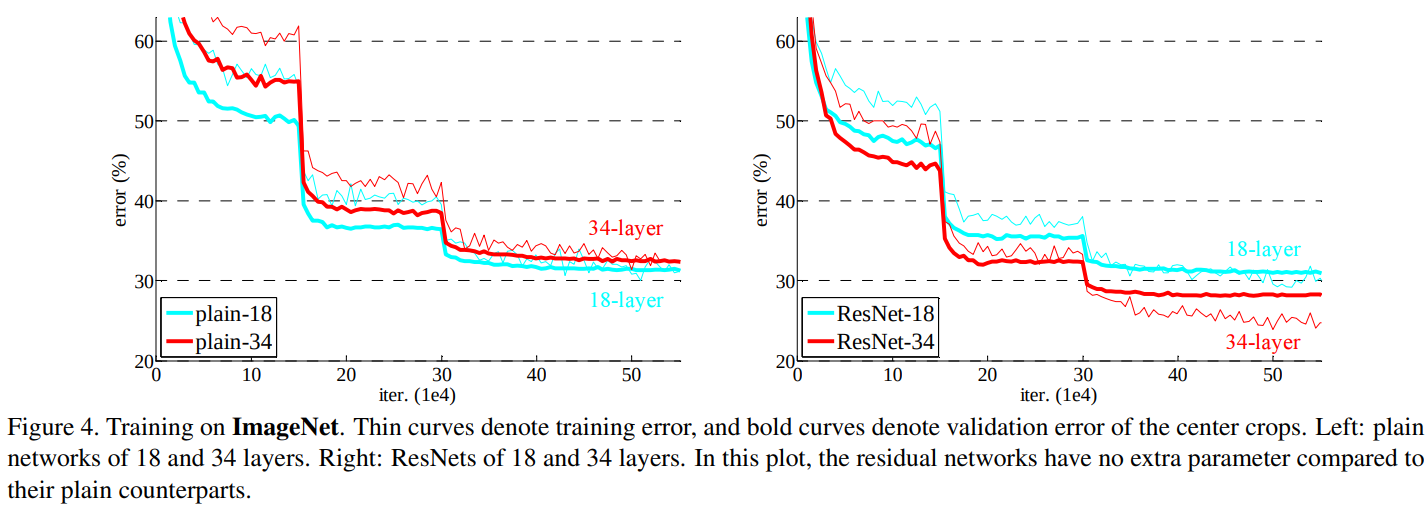# 2016-CVPR-Deep Residual Learning for Image Recognition

## 2. 框架## 3. 实验## 4. 分析

• 第一层：$\frac{\partial [f(h(\boldsymbol{x})) + h(\boldsymbol{x})]}{\partial \boldsymbol{x}} = \frac{\partial f(h(\boldsymbol{x}))}{\partial \boldsymbol{x}} + \frac{\partial h(\boldsymbol{x})}{\partial \boldsymbol{x}}$
• 第二层：$\frac{\partial [g(f(h(\boldsymbol{x})) + h(\boldsymbol{x})) + f(h(\boldsymbol{x})) + h(\boldsymbol{x})]}{\partial \boldsymbol{x}} = \frac{\partial g(f(h(\boldsymbol{x})) + h(\boldsymbol{x}))}{\partial \boldsymbol{x}} + \frac{\partial f(h(\boldsymbol{x}))}{\partial \boldsymbol{x}} + \frac{\partial h(\boldsymbol{x})}{\partial \boldsymbol{x}}$

## 附录

1. He, K., Zhang, X., Ren, S., & Sun, J. (2016). Deep residual learning for image recognition. In Proceedings of the IEEE conference on computer vision and pattern recognition (pp. 770-778).